# Formatting - Time with Two Digit Minutes

Wikitechy | 2767 Views | javascript | 05 Jun 2016

• JavaScript is a loosely-type language, which means that the data type of a variable can be easily changed.
• Hence, the same variable storing a number can be made to store a string value.
• Here we can add a blank string to curr_min variable. This operation converts the data type of this variable from number to a string.
• Using the length property of the string object, we can then check the number of digits.
• If the number of digits are one, a leading zero is attached to the variable.
• To add  leading zero, we first have to check the value returned from getMinutes(). We then convert this numeric value to a string and check its length. If the length is 1, we need to add the leading zero.

#### Sample Code :

```<script type="text/javascript">
var AM_PM_Variable = "";
var date_var = new Date();
var curr_hour = date_var.getHours();
if (curr_hour < 12)
{
AM_PM_Variable = "AM";
}
else
{
AM_PM_Variable = "PM";
}
if (curr_hour == 0)
{
curr_hour = 12;
}
if (curr_hour > 12)
{
curr_hour = curr_hour - 12;
}
var curr_min = date_var.getMinutes();
if (curr_min.length == 1)
{
curr_min = "0" + curr_min;
}
document.write(curr_hour + " : " + curr_min + " " + AM_PM_Variable);
</script>
```

#### Code Explanation :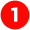Here in this statement, a variable AM_PM_Variable is initialized. Depending upon the value of curr_hour, we assign AM or PM to this variable.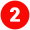Here in this statement we create an object for the date function using new operator and the object name as “date_var”. So, the current date will be stored in the variable “date_var”.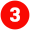In this statement we get the current hour by using the getHours function with the reference of “date_var” object and the current hour’s value will be stored in the variable “curr_hour”.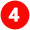Here we check the current hour is less than “12” are not. Apart from that if the current hour is less than 12 means set the variable AM_PM_Variable as “AM” otherwise set “PM”.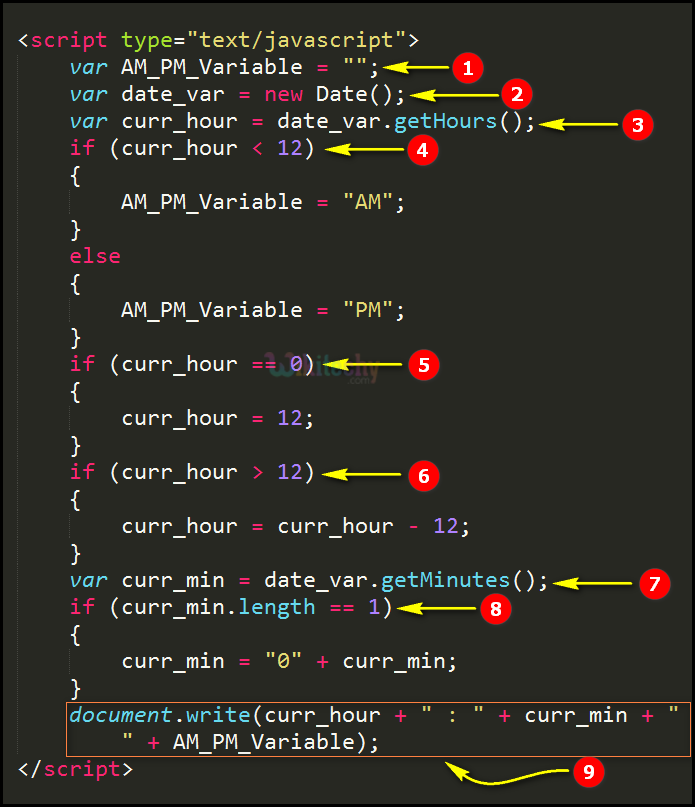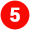Here in this statement we have to check the current hour is equal to “0” are not. If the current hour is equal to “0” set curr_ hour variable as “12”.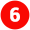In this statement if curr_hour is more than 12 we subtract 12 from it, in order to convert the hours to 12-hour format.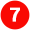In this statement we get the current hour by using the getMinutes function with the reference of “date_var” object and the current minute’s value will be stored in the variable “curr_min”.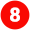Here we check the current minutes of time length is 1 or not if the length is equal to “1” means add “0” to the curr_minutes.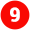Here in this statement “document.write” function is used to write the current hours, current minutes & AM (or) PM in the browser window by using the concatenation operator” +”.

#### Sample Output :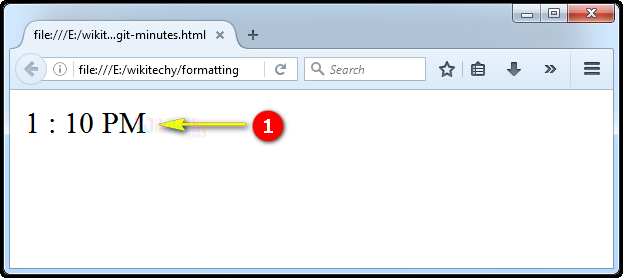Here in this output we display the current time as “1:10 PM” which is one hours and ten minutes PM (Post Meridiem) as shown.

Bug Bounty
Webinar